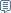### 首页 > 线缆设计|参数计算

#### [问题求助 ] 载流量的计算

##### lishi#### RTV - room temperature vulcanizing[zation]室温硫化 点赞（1）投诉

##### leopan##### P:2006-06-21 19:49:46 2

CableExpert V5.0下载请到:http://www.cable168.com

#### Cabling Factor - 集合系数。用于计算电缆集合后之外径 点赞（1）投诉

##### yjlw03#### semi-patented rod - 半淬火线杆 点赞（0）投诉

##### leopan##### P:2006-06-23 17:48:10 4yjlw03:

#### all-plastic power cable - 全塑料电力电缆 点赞（0）投诉

##### yjlw03#### dielectric withstanding tester - 耐电压试验机 点赞（0）投诉

##### leopan#### rolling equipment - 轧制设备,压延设备 点赞（0）投诉

##### lishi#### vulcanized bitumen-sheathed cable - 硫化沥青护套电缆 点赞（0）投诉

##### yjlw03#### access network - 接入网 点赞（0）投诉

##### leopan##### P:2006-06-26 21:42:24 9

1.導體交流電阻: R=R’(1+YS+YP)

1.1 最高工作溫度下導體直流電阻  R’=R0*[1+ a20(θ-20)]         a20=0.00393

=0.146*[1+ 0.00393*(90-20)] =0.1862  OHM/KM

=0.0001862 OHM/M

1.2集膚效應因數：

Xs2=8πf ×10-7Ks/R’      Ks=1

=8*3.1416*50*10-7/0.0001862

=0.674886

Xs4=0.455471

Ys=Xs4/(192+0.8Xs4)=0.455471/(192+0.8*0.455471)=0.0023678

*软件计算结果为:0.0023687  因软件计算中为计算到结果才进行一定位数的舍取,计算过程中都是按能计算的最大位数,所以更精确.而手工计算中间过程也只能取有限的小数位数,所以有一些较小的差异.(下同)

1.3三芯或三根單芯電纜佈設的鄰近效應因數：

YP=XP4 ×（dC/s2×{0.312×（dC/s2 + 1.18/[Xp4/(192+0.8Xp4) ]} /(192+0.8XP4)

XP2=8πf×10-7KP/R’ =0.674886 (如上)  KP=1 不乾燥浸漬緊壓及非緊壓絞合導體KP均為1.0

XP 4=0.455471

dC/s2=(13.5/39.4)2=0.117402

YP=XP4 ×（dC/s2×{0.312×（dC/s2 + 1.18/{[Xp4/(192+0.8Xp4) ]+0.27}} /(192+0.8XP4)

=0.05347321×[0.035787+1.18/(0.0023678+0.27)]/192.36438

=0.001419

*软件计算结果为:0.001215

1.4  非钢管中导体90度交流电阻:

R=R’(1+YS+YP)= 0.0001862*(1+0.0023678+0.001419)=0.0001868 OHM/M=0.1868 ohm/km

1.5  钢管中导体90度交流电阻:

R=R’[1+1.5(YS+YP)]= 0.0001862*[1+1.5*(0.0023678+0.001419)]

=0.0001873 OHM/M=0.1873 ohm/km

2.电缆本体热阻

2.1 绝缘热阻:

=3.5*
ln(1+2*2/13.5)/6.28319=0.144559

2.2 外被热阻:

T3=ρT* ln (1+2t3/D’a) / (2π)   ρT=5.0   t3=1.25    D’a =17.2

=[5*ln(1+2*1.25/17.2)]/6.283185=0.107994

2.3 电缆槽布设外部热阻 :  S=2D

T4=1/ [π*De*h*(△θS)1/4 ]

h：散熱係數  h=Z/Deg +E   De单位为米

h=Z/Deg +E

h=0.21/(0.0197)0.6+3.94=0.21/0.0947719+3.94=6.15585

T4=1/ [π*De*h*(△θS)1/4 ]

=1/[3.141593*0.0197*6.15585*(△θS)1/4 ]      (△θS)1/4=2.52

=1/[0.38098175*(△θS)1/4]=1.041585

:(△θS)1/4无法进行手动计算,由计算机编程迭代算出.

2.4  土壤中布设外部热阻 : S=2D

T4=Pt*{ln[u+(u2-1)1/2 ]+ln[1+(2L/S1)2]}/(2π)

Pt即土壤热阻系数1.0

u=2L/D=2000/19.7=101.5228           L埋地深度 :1000   D :电缆外径 :19.7

s1相邻电缆间轴心距离 2*19.7=39.4 mm]

T4=1.0{ln[(101.5228+(101.52282-1)1/2)+ln[1+(2000/39.4)2]]/6.283185

=(5.313406+7.85466)/6.283185=2.095763

2.5   钢管中布设外部热阻 :

T4=T’4+T’’4+T’’’4

2.5.1 电缆表面和管道内表面之间的空气热阻 :

T’4=U/[1+0.1(V+Yθm)*De]

θm­­：電纜與管道之間的介質平均溫度  請確認：θm：建議XLPE電纜取30℃（即假定電纜護套溫度為60，管道溫度為30）；PE耐火電纜取 15℃（即假定電纜護套溫度為45，管道溫度為30

U,V,Y取值为:5.2   1.4   0.011  θm=30   De=19.7

T’4=5.2/[1+0.1(1.4+0.011*30)*19.7]=1.179647

2.5.2  钢管本体热阻忽略

2.5.3  管道外部热阻:( 钢管及PVC)

<<电线电缆手册>>P343页中的镜象法:

T’’’4=PT4*ln(4*L*Fe/Dg)    Dg=为管道外径   PT4=1.0   L=1000 (埋地深度)

#### A wire - 地址线 点赞（0）投诉

##### leopan##### P:2006-06-26 21:43:51 10

Dg：管道外徑  （等於管道內徑+2倍管道厚度）

Dd：管道內徑 1.5倍電纜外徑計算；管道厚度按 0.06倍管道內徑計算

:Dg=1.5*19.7+2*0.06*(1.5*19.7)=33.1 mm

43条布设(按最左下角一条计算):

Fe=(2200/200)*(2409/200)=132.5

T’’’4=1.0*ln(4*1000*132.5/33.1)/6.283185=1.541

66(按最左下角一条计算):

* 66条的布设方式中,由于埋地电缆间被土壤填充,相互间的热影响理论计算与实际有很大差异,因此66孔的电缆布设选择最左下角一条电缆(即载流量处于三条电缆载流量中间值的电缆)进行计算,这个计算值在实际工作状态与中间电缆的效应基本符合.(如果按中间电缆计算,其电缆安全电流仅为257A)

T’’’4= PT4*ln(4*L*Fe/Dg)/(2π)      PT4为土壤热阻系数:1.0

=1.0*ln(4*1000*54326/33.1)/6.28315

=2.49831

4
3:  T4=T4’+T4’’+T4’’’=1.179647+1.541=2.720647

66:  T4=T4’+T4’’+T4’’’=1.179647+2.49831=3.677957

2.6  pvc管中布设外部热阻 :

T4=T’4+T’’4+T’’’4

2.6.1 电缆表面和管道内表面之间的空气热阻 :

T’4=U/[1+0.1(V+Yθm)*De]

θm­­：電纜與管道之間的介質平均溫度  請確認：θm：建議XLPE電纜取30℃（即假定電纜護套溫度為60，管道溫度為30）；PE耐火電纜取 15℃（即假定電纜護套溫度為45，管道溫度為30

U,V,Y取值为:5.2   0.91   0.01  θm=30   De=19.7

T’4=5.2/[1+0.1(0.91+0.01*30)*19.7]=1.536779

2.6.2  PVC管本体热阻:

T’’4=ρT*ln(Dg/Dd)/(2π)   PT=6.0  pvc管热阻   Dg=33.1   Dd=1.5*19.7=29.6

=6.0*ln(33.1/29.6)/6.28315=0.1067225

2.6.3  管道外部热阻:

PVC管中布设电缆外部热阻:
4
3:  T4=T4’+T4’’+T4’’’=1.536779+0.1067225+1.541=3.1845

66:  T4=T4’+T4’’+T4’’’=1.536779+0.1067225+2.49831=4.1418

I={θ/[ nT1+NR(1+λ1)T2+NR(1+λ1+λ2)(T3+T4)]}1/2

θ=90-40=50

R=0.1868 OHM/KM=0.0001868  OHM/M

={50/[0.0001868*0.144559+0.0001868*(0.107994+1.041585)]}2

=[50/(0.0000270036212+0.0002147413572)]1/2

=(50/0.0002417449784)^2

=455  A

θ=90-25=65

I={θ/[RT1 +R (T3+T4)]}1/2

={65/[0.0001868*0.144559+0.0001868*(0.107994+2.095763)]}2

=[65/(0.0000270036212+0.000411644996)]1/2

=385  A

43条金属管  :

θ=90-25=65

I={θ/[RT1 +R (T3+T4)]}1/2

={65/[0.0001873*0.144559+0.0001873*(0.107994+2.720647)]}2

=[65/(0.0000270759007+0.000411644996)]1/2

=342 A

66条金属管道 :

θ=90-25=65

I={θ/[RT1 +R (T3+T4)]}1/2

={65/[0.0001873*0.144559+0.0001873*(0.107994+3.677957)]}2

=297  A

43PVC管道 :

θ=90-25=65

I={θ/[RT1 +R (T3+T4)]}1/2

={65/[0.0001868*0.144559+0.0001868*(0.107994+3.1845)]}2

=318 A

66PVC管道 :

θ=90-25=65

I={θ/[RT1 +R (T3+T4)]}1/2

={65/[0.0001868*0.144559+0.0001868*(0.107994+4.1418)]}2

=281  A

#### Tempmaster - 一种高频感应预热器(瑞士Zumbach Electronic AG公司产品商品名,用于预热数据传输线及通信线,加热速度为6000次循环/秒) 点赞（0）投诉

##### 管理员#### continuous strand annealing - (线材的)多根连续退火 点赞（0）投诉

##### 山人#### optical fibre transmission line - 光纤传输线 点赞（0）投诉

##### chuanweilu#### centreless grinding and polishing machine - 无心研磨抛光机 点赞（0）投诉

##### luyuxue1983##### P:2006-07-22 11:11:43 14

非常感谢leopan以及各位同仁

#### rod - 杆,线杆,棒材 点赞（0）投诉

##### yjlw03#### TS - 表示两芯橡胶绝缘及被覆铜箔丝导体花线。用于轻任型 50W 以下之电气器具用线，亦可用于潮湿场所，每条长度为 8 呎以下。 点赞（2）投诉

##### 163